# TORSION OF SHAFTSReading time: 1 minute

When a cylindrical shaft is subjected to equal and opposite couples at the ends, either it will be in equilibrium or it will rotate at a uniform rate. In either case, it is subjected to torsion and the stresses set up by every cross-section are shear stresses. At any point in the cross-section of a shaft, there is a state of simple shear, the two planes of shear, that is the planes across which the stress is wholly tangential being the cross-section itself and the plane through the point and the axis of the shaft.

Assumptions:

In deriving the torsion formula, the following assumptions are made:

a) Circular sections remain circular.

b) Plane sections remain plane and do not warp.

c) The projection upon a transverse section of straight radial lines in the section remains straight.

d) The shaft is loaded by twisting couples in planes that are perpendicular to the axis of the shaft.

e) Stresses do not exceed the proportional limit.

f) The material of the bar is homogeneous and perfectly elastic, and obeys Hooke’s Law.

Torsion Formula: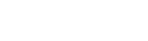Where q = shear intensity at radius r

r = radius at a point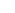= maximum shear stress at the surface of a shaft

R = radius of the shaft

G = shear modulus of the material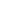= angle of twist

l = length of the shaft

T = torque

J = polar moment of inertia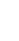is also termed as twist per unit length.

The expression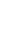may be called the torsional section modulus of the section and is analogous to the section modulus found in the flexure formula. The term GJ is called torsional rigidity. The angle of twist in a shaft under torsion corresponds to deflection of a beam under transverse loading and is an indication of the stiffness of the shaft.

To ensure adequate stiffness of a shaft, the angle of twist is usually limited to about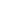in a length of 20 diameters.

Polar Moment of Inertia:

J = 2I

For a solid circular section of diameter D,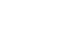For a hollow circular section of external diameter D and internal diameter d,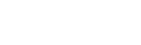Solid Shaft

Maximum shear stress,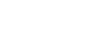Angle of twist,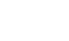Where T = twisting moment

d = diameter of the shaft

Hollow Shaft:

Maximum shear stress,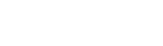Where T = twisting moment

D = external diameter of shaft and

d = internal diameter of shaft